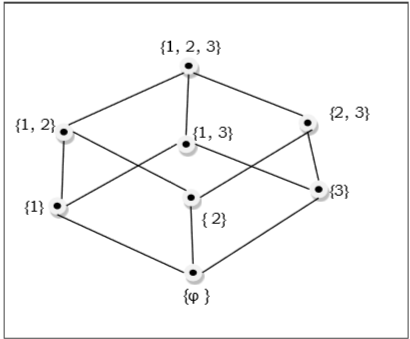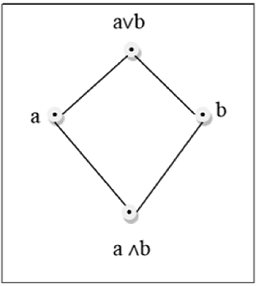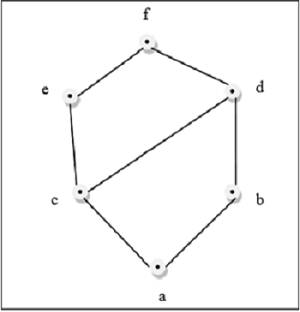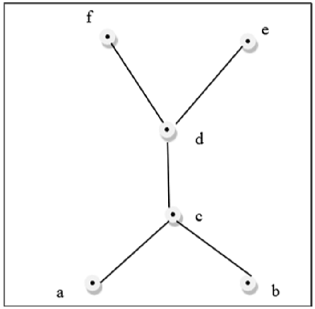# Discrete Mathematics - Group Theory

## Semigroup

A finite or infinite set $‘S’$ with a binary operation $‘\omicron’$ (Composition) is called semigroup if it holds following two conditions simultaneously −

• Closure − For every pair $(a, b) \in S, \:(a \omicron b)$ has to be present in the set $S$.

• Associative − For every element $a, b, c \in S, (a \omicron b) \omicron c = a \omicron (b \omicron c)$ must hold.

### Example

The set of positive integers (excluding zero) with addition operation is a semigroup. For example, $S = \lbrace 1, 2, 3, \dots \rbrace$

Here closure property holds as for every pair $(a, b) \in S, (a + b)$ is present in the set S. For example, $1 + 2 = 3 \in S]$

Associative property also holds for every element $a, b, c \in S, (a + b) + c = a + (b + c)$. For example, $(1 + 2) + 3 = 1 + (2 + 3) = 5$

## Monoid

A monoid is a semigroup with an identity element. The identity element (denoted by $e$ or E) of a set S is an element such that $(a \omicron e) = a$, for every element $a \in S$. An identity element is also called a unit element. So, a monoid holds three properties simultaneously − Closure, Associative, Identity element.

### Example

The set of positive integers (excluding zero) with multiplication operation is a monoid. $S = \lbrace 1, 2, 3, \dots \rbrace$

Here closure property holds as for every pair $(a, b) \in S, (a \times b)$ is present in the set S. [For example, $1 \times 2 = 2 \in S$ and so on]

Associative property also holds for every element $a, b, c \in S, (a \times b) \times c = a \times (b \times c)$ [For example, $(1 \times 2) \times 3 = 1 \times (2 \times 3) = 6$ and so on]

Identity property also holds for every element $a \in S, (a \times e) = a$ [For example, $(2 \times 1) = 2, (3 \times 1) = 3$ and so on]. Here identity element is 1.

## Group

A group is a monoid with an inverse element. The inverse element (denoted by I) of a set S is an element such that $(a \omicron I) = (I \omicron a) = a$, for each element $a \in S$. So, a group holds four properties simultaneously - i) Closure, ii) Associative, iii) Identity element, iv) Inverse element. The order of a group G is the number of elements in G and the order of an element in a group is the least positive integer n such that an is the identity element of that group G.

### Examples

The set of $N \times N$ non-singular matrices form a group under matrix multiplication operation.

The product of two $N \times N$ non-singular matrices is also an $N \times N$ non-singular matrix which holds closure property.

Matrix multiplication itself is associative. Hence, associative property holds.

The set of $N \times N$ non-singular matrices contains the identity matrix holding the identity element property.

As all the matrices are non-singular they all have inverse elements which are also nonsingular matrices. Hence, inverse property also holds.

## Abelian Group

An abelian group G is a group for which the element pair $(a,b) \in G$ always holds commutative law. So, a group holds five properties simultaneously - i) Closure, ii) Associative, iii) Identity element, iv) Inverse element, v) Commutative.

### Example

The set of positive integers (including zero) with addition operation is an abelian group. $G = \lbrace 0, 1, 2, 3, \dots \rbrace$

Here closure property holds as for every pair $(a, b) \in S, (a + b)$ is present in the set S. [For example, $1 + 2 = 2 \in S$ and so on]

Associative property also holds for every element $a, b, c \in S, (a + b) + c = a + (b + c)$ [For example, $(1 +2) + 3 = 1 + (2 + 3) = 6$ and so on]

Identity property also holds for every element $a \in S, (a \times e) = a$ [For example, $(2 \times 1) = 2, (3 \times 1) = 3$ and so on]. Here, identity element is 1.

Commutative property also holds for every element $a \in S, (a \times b) = (b \times a)$ [For example, $(2 \times 3) = (3 \times 2) = 3$ and so on]

## Cyclic Group and Subgroup

A cyclic group is a group that can be generated by a single element. Every element of a cyclic group is a power of some specific element which is called a generator. A cyclic group can be generated by a generator ‘g’, such that every other element of the group can be written as a power of the generator ‘g’.

### Example

The set of complex numbers $\lbrace 1,-1, i, -i \rbrace$ under multiplication operation is a cyclic group.

There are two generators − $i$ and $–i$ as $i^1 = i, i^2 = -1, i^3 = -i, i^4 = 1$ and also $(–i)^1 = -i, (–i)^2 = -1, (–i)^3 = i, (–i)^4 = 1$ which covers all the elements of the group. Hence, it is a cyclic group.

Note − A cyclic group is always an abelian group but not every abelian group is a cyclic group. The rational numbers under addition is not cyclic but is abelian.

A subgroup H is a subset of a group G (denoted by $H ≤ G$) if it satisfies the four properties simultaneously − Closure, Associative, Identity element, and Inverse.

A subgroup H of a group G that does not include the whole group G is called a proper subgroup (Denoted by $H < G$). A subgroup of a cyclic group is cyclic and a abelian subgroup is also abelian.

### Example

Let a group $G = \lbrace 1, i, -1, -i \rbrace$

Then some subgroups are $H_1 = \lbrace 1 \rbrace, H_2 = \lbrace 1,-1 \rbrace$,

This is not a subgroup − $H_3 = \lbrace 1, i \rbrace$ because that $(i)^{-1} = -i$ is not in $H_3$

## Partially Ordered Set (POSET)

A partially ordered set consists of a set with a binary relation which is reflexive, antisymmetric and transitive. "Partially ordered set" is abbreviated as POSET.

### Examples

• The set of real numbers under binary operation less than or equal to $(\le)$ is a poset.

Let the set $S = \lbrace 1, 2, 3 \rbrace$ and the operation is $\le$

The relations will be $\lbrace(1, 1), (2, 2), (3, 3), (1, 2), (1, 3), (2, 3)\rbrace$

This relation R is reflexive as $\lbrace (1, 1), (2, 2), (3, 3)\rbrace \in R$

This relation R is anti-symmetric, as

$\lbrace (1, 2), (1, 3), (2, 3) \rbrace \in R\ and\ \lbrace (1, 2), (1, 3), (2, 3) \rbrace ∉ R$

This relation R is also transitive as $\lbrace (1,2), (2,3), (1,3)\rbrace \in R$.

Hence, it is a poset.

• The vertex set of a directed acyclic graph under the operation ‘reachability’ is a poset.

## Hasse Diagram

The Hasse diagram of a poset is the directed graph whose vertices are the element of that poset and the arcs covers the pairs (x, y) in the poset. If in the poset $x < y$, then the point x appears lower than the point y in the Hasse diagram. If $x<y<z$ in the poset, then the arrow is not shown between x and z as it is implicit.

### Example

The poset of subsets of $\lbrace 1, 2, 3 \rbrace = \lbrace \emptyset, \lbrace 1 \rbrace, \lbrace 2 \rbrace, \lbrace 3 \rbrace, \lbrace 1, 2 \rbrace, \lbrace 1, 3 \rbrace, \lbrace 2, 3 \rbrace, \lbrace 1, 2, 3 \rbrace \rbrace$ is shown by the following Hasse diagram −## Linearly Ordered Set

A Linearly ordered set or Total ordered set is a partial order set in which every pair of element is comparable. The elements $a, b \in S$ are said to be comparable if either $a \le b$ or $b \le a$ holds. Trichotomy law defines this total ordered set. A totally ordered set can be defined as a distributive lattice having the property $\lbrace a \lor b, a \land b \rbrace = \lbrace a, b \rbrace$ for all values of a and b in set S.

### Example

The powerset of $\lbrace a, b \rbrace$ ordered by \subseteq is a totally ordered set as all the elements of the power set $P = \lbrace \emptyset, \lbrace a \rbrace, \lbrace b \rbrace, \lbrace a, b\rbrace \rbrace$ are comparable.

### Example of non-total order set

A set $S = \lbrace 1, 2, 3, 4, 5, 6 \rbrace$ under operation x divides y is not a total ordered set.

Here, for all $(x, y) \in S, x | y$ have to hold but it is not true that 2 | 3, as 2 does not divide 3 or 3 does not divide 2. Hence, it is not a total ordered set.

## Lattice

A lattice is a poset $(L, \le)$ for which every pair $\lbrace a, b \rbrace \in L$ has a least upper bound (denoted by $a \lor b$) and a greatest lower bound (denoted by $a \land b$). LUB $(\lbrace a,b \rbrace)$ is called the join of a and b. GLB $(\lbrace a,b \rbrace )$ is called the meet of a and b.### ExampleThis above figure is a lattice because for every pair $\lbrace a, b \rbrace \in L$, a GLB and a LUB exists.This above figure is a not a lattice because $GLB (a, b)$ and $LUB (e, f)$ does not exist.

Some other lattices are discussed below −

### Bounded Lattice

A lattice L becomes a bounded lattice if it has a greatest element 1 and a least element 0.

### Complemented Lattice

A lattice L becomes a complemented lattice if it is a bounded lattice and if every element in the lattice has a complement. An element x has a complement x’ if $\exists x(x \land x’=0 and x \lor x’ = 1)$

### Distributive Lattice

If a lattice satisfies the following two distribute properties, it is called a distributive lattice.

• $a \lor (b \land c) = (a \lor b) \land (a \lor c)$

• $a \land (b \lor c) = (a \land b) \lor (a \land c)$

### Modular Lattice

If a lattice satisfies the following property, it is called modular lattice.

$a \land( b \lor (a \land d)) = (a \land b) \lor (a \land d)$

## Properties of Lattices

### Idempotent Properties

• $a \lor a = a$

• $a \land a = a$

### Absorption Properties

• $a \lor (a \land b) = a$

• $a \land (a \lor b) = a$

### Commutative Properties

• $a \lor b = b \lor a$

• $a \land b = b \land a$

### Associative Properties

• $a \lor (b \lor c) = (a \lor b) \lor c$

• $a \land (b \land c) = (a \land b) \land c$

## Dual of a Lattice

The dual of a lattice is obtained by interchanging the '$\lor$' and '$\land$' operations.

### Example

The dual of $\lbrack a \lor (b \land c) \rbrack\ is\ \lbrack a \land (b \lor c) \rbrack$